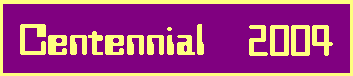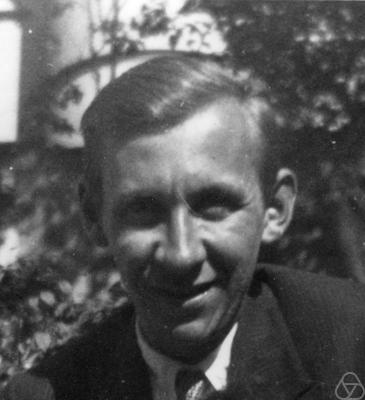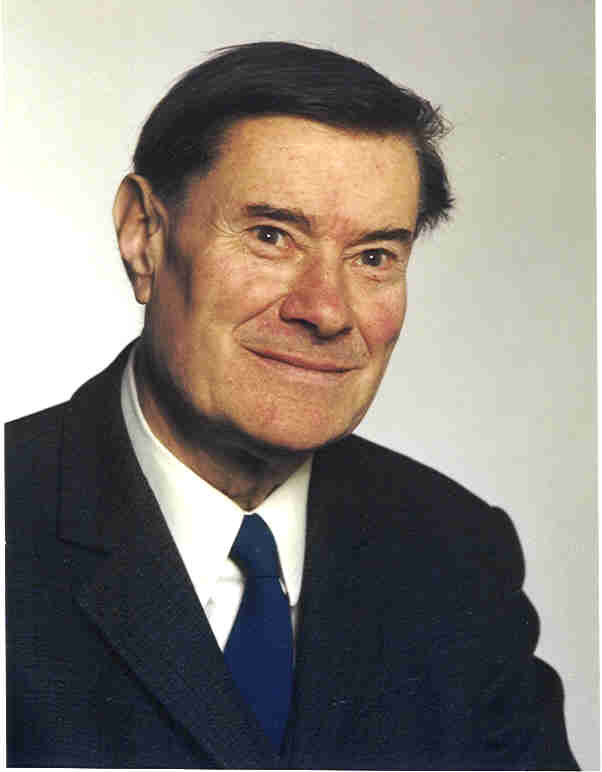# 2-Stage Metabelian 3-Groups

## 1. Definition of Principalization Types.

Let K be a base field with 3-class rank 2 and
with 3-class group Syl3(C(K)) of 3-elementary abelian type (3,3).
Denote by K1 the 1st Hilbert 3-class field of K.
According to the Artin Reciprocity Law of Class Field Theory,
the 3-class group Syl3(C(K)) = (3,3) of K is isomorphic to the
relative automorphism group Gal(K1|K) of K1 over K.
In particular, the 4 cyclic subgroups Ci (1 <= i <= 4) of Syl3(C(K)) are mapped to the
Galois groups Gal(K1|Ni) = Mi of 4 unramified cyclic cubic extensions Ni of K.
In fact, the Ci = Norm(Ni|K)Syl3(C(Ni)) are relative norms of 3-class groups.
The following diagrams illustrate the Galois correspondence and the Artin isomorphism:

 K1 = N0 / / \ \ N1 N2 N3 N4 \ \ / / K
 Gal(K1|K1) = 1 / | \ M1 Gal(K1|Ni) = Mi M4 \ | / Gal(K1|K)
 1 / / \ \ C1 C2 C3 C4 \ \ / / Syl3(C(K)) = C0

Now we consider the class extension homomorphisms j(Ni|K): Syl3(C(K)) --> Syl3(C(Ni)).
We say an ideal class of K that is mapped to the principal class 1 of Ni by j(Ni|K)
principalizes or becomes principal or capitulates in Ni.

According to Hilbert's Theorem 94, none of the principalization kernels Ker j(Ni|K) is trivial,
since there is an isomorphism to the unit cohomology of N = Ni:
Ker j(N|K) = PN < S > /PK = EN|K/UNS-1 != 1,
where Gal(N|K) = < S > and EN|K denotes the intersection of UN with Ker Norm(N|K).

According to the Hilbert/Artin/Furtwängler Principal Ideal Theorem,
we have complete principalization in the Hilbert 3-class field K1 = N0:
Ker j(N0|K) = Syl3(C(K)) = C0.

Thus, there are 5 possibilities for each Ker j(Ni|K), C0,...,C4,
and if K is a quadratic field we define a Natural Principalization Type (k(1),...,k(4)),
ordering the Ni (1 <= i <= 4),which have dihedral absolute groups Gal(Ni|Q) = D(6),
by increasing regulators of their absolute cubic subfields Li:
for each index 0 <= i <= 4 there exists a unique index 0 <= k(i) <= 4
such that Ker j(Ni|K) = Ck(i) (in particular, always k(0) = 0).

An Important Application as Example.

In the following tables, we give the natural principalization type (k(1)...k(4))
of all imaginary quadratic fields K = Q(d1/2) with discriminant -50000 < d < 0 and 3-class group of type (3,3).
These recent computations of 2003 for 42 new cases in the range -50000 < d < -30000 
extend our own results for 22 fields with -30000 < d < -20000 of 1989 
and the 13 examples with -20000 < d < 0 of Heider and Schmithals in 1982  .
As a supplement, we give some singular cases
with associated 2-stage metabelian 3-groups of exceptionally high order
in the range -200000 < d < -50000  .
Exceptional cases are printed in boldface.

No.dK(k(1)...k(4))TypeRef.
1-3896(4111)H.4
2-4027(2331)D.10
3-6583(4111)H.4
4-8751(1421)D.10
5-9748(2214)E.9
6-12067(4321)G.19
7-12131(2244)D.5
8-15544(1122)E.6
9-16627(2313)E.14
10-17131(3214)G.16
11-18555(1313)E.6
12-19187(4334)D.5
13-19651(1312)D.10
14-20276(3232)D.5
15-20568(1414)D.5
16-21224(1421)D.10
17-21668(2111)H.4.V1[5,7]
18-22395(1132)E.9
19-22443(2214)E.9
20-22711(2331)D.10
21-23428(3323)H.4
22-23683(3223)E.6
23-24340(1133)D.5
24-24884(2134)G.16
25-24904(2241)D.10
26-25447(3343)H.4
27-26139(3221)D.10
28-26760(1133)D.5
29-27156(4221)F.11
30-27355(4441)H.4
31-27640(1332)E.9
32-27991(2122)H.4
33-28031(4332)D.10
34-28279(1432)G.16
35-28759(2414)D.10
36-31271(4112)E.14
37-31639(1331)D.5
38-31908(3211)F.12
39-31999(1133)D.5
40-32968(3232)D.5
41-34027(3313)H.4.V1
42-34088(4212)D.10
43-34507(4334)D.5
44-34867(1134)E.8
45-35367(4224)D.5
46-35539(4231)G.16
No.dK(k(1)...k(4))TypeRef.
47-36276(4442)H.4
48-36807(4212)D.10
49-37219(3343)H.4
50-37540(4442)H.4
51-37988(3231)E.9
52-39736(4134)E.9
53-39819(3343)H.4
54-40299(2241)D.10
55-40692(2414)D.10
56-41015(1312)D.10
57-41063(2422)H.4
58-41583(1221)D.5
59-41671(1331)D.5
60-42423(4332)D.10
61-42619(3234)E.8
62-42859(4313)E.14
63-43192(2213)D.10
64-43307(2244)D.5
65-43827(3313)H.4
66-43847(2311)E.14
67-44004(3431)D.10
68-45835(2414)D.10
69-45887(3244)E.9
70-46551(2122)H.4
71-46587(4223)D.10
72-48052(1312)D.10
73-48472(4134)E.9
74-48667(2231)E.9
75-49128(4133)D.10
76-49812(3242)D.10
77-49924(3412)G.19
---------------------------
106-67480(2343)F.13
147-96827(2143)G.19.V
166-104627(3442)F.13
210-124363(4411)F.7
235-135059(1243)G.16.V
236-135587(1422)F.12
261-156452(4321)G.19.V
268-159208(2343)F.13.V
271-160403(3314)F.12
288-167064(2343)F.13
314-184132(4211)F.12
317-185747(1243)G.16.V
320-186483(3343)H.4.V2
330-189959(1323)F.12

## 2. The Connection between Class, Order, and Commutator Subgroup.

In the preceding section and in our previous communication,
concerning the descending central series of 2-stage metabelian 3-groups,
we published several top recent results (discovered between January and August, 2003) for quadratic fields K
whose Sylow 3-subgroup Syl3(C(K)) of the class group C(K), i. e., the 3-class group, is of type (3,3).

We denoted by G = G1 > G2 > ... > Gi > ... > Gm = 1 the descending central series of class m - 1 of G with Gi+1 = [Gi,G].
In particular, G2 = [G,G] is the commutator subgroup G' of G.

Now it is adequate to give the solution of a very fundamental problem:
How do we get the class m-1 and the order 3n
of the 2-stage metabelian 3-group G = G(K2|K) in ZEF(m,n) of the 2nd Hilbert 3-class field K2
of an arbitrary base field K with 3-class group Syl3(C(K)) = (3,3) over K
from the structure of the (abelian) commutator subgroup
G' = G2 = Gal(K2|K1) = Syl3(C(K1))
which coincides with the 3-class group of the 1st Hilbert 3-class field K1 of K:

1. There are bounds for the order in dependence on the class:
m <= n <= 2m - 3

2. Since there are only upper triangular entries in an (m,n)-diagram, we use an ordering parameter
e = n + 2 - m ( >= 2 )
to measure the distance from the diagonal ( e = 2 )

3. Then we have an almost unique correspondence between the structure of G' and the pair (m,n), expressed in the following (easily extensible) table .
Only the red pairs (m,n) are exceptional, since they correspond to 2 possible structures of G'.

In particular, we get 3 theorems concerning important cases:

THEOREM 1. G is of maximal class ( n = m, resp. e = 2 ) <==> the 3-rank of G' is r <= 2

THEOREM 2. G is of second maximal class ( n = m + 1, resp. e = 3 ) <==> the 3-rank of G' is r = 3 or G' = (3,3,3,3)

THEOREM 3. G is of lower than second maximal class ( n >= m + 2, resp. e >= 4 ) <==> the 3-rank of G' is r = 4 and the order of G' is >= 243

## 3. The Connection between Principalization Types, Class, and Order.

The next question is: How are the principalization types related to the parameter e and the pairs (m,n) ?

The fine (necessary and sufficient) conditions have been determined by Brigitte Nebelung  .

Here, we only give a coarse connection:
Denote by 0 <= f <= 4 the number of those unramified cubic extensions of K
where the full 3-class group Syl_3(C(K)) = (3,3) of K becomes principal (i. e., capitulates).

Then we have 2 theorems:

THEOREM (i) G is of maximal class ( n = m, resp. e = 2 ) <==>
f = 4, i. e., principalization type (0,0,0,0) or
f = 3, i. e., principalization type (1,0,0,0) or (2,0,0,0) or
f = 0 and the principalization type is (1,1,1,1) (which implies n = 3 )

THEOREM (ii) G is of second maximal class or lower ( n >= m + 1, resp. e >= 3 ) <==>
f <= 2 but the principalization type is different from (1,1,1,1)

## 4. Peculiarities of Quadratic Fields.

The last question is, what happens, if K is a quadratic field ?

Well, according to Scholz and Taussky  we have 2 theorems:

THEOREM 1. Principalization type (1,1,1,1) (denoted as Type "A" by S. & T.) is impossible for quadratic fields K

THEOREM 2. f = 0 for any imaginary quadratic field K

Example: d = -28031 ==> f = 0 and p.t. != (1,1,1,1) ==> e != 2 ==> r >= 3 ==> G' != (9,3). In fact, G' = (3,3,3).

## 5. Summary of recently (2003) discovered connections.

In general, Nebelung's results still admit a wide range of 2-stage metabelian 3-groups G = G(K2 | K)
for the 2nd Hilbert 3-class field K2 of an algebraic number field K
with 3-class group Syl3(C(K)) of type (3,3)
and with a certain principalization type (k(1),...,k(4)) in the four unramified cyclic cubic extensions N1,...,N4 of K.

However, our concrete numerical results  discovered in 2003 for quadratic base fields K show that, in this special case,
a principalization type (k(1),...,k(4)) uniquely determines the class m-1 and order 3n of G = G(K2 | K),
except in the sections "F","G", and "H", where two possibilities arise.
(Section "A" is impossible for quadratic base fields K and
sections "B" and "C" cannot occur at all, for group theoretic reasons.)

In the following table, the types (k(1),...,k(4))) are arranged into sections, according to [1,4] ,
and they are numbered similarly as in  .
We always give a canonical representative (CR) of the type's equivalence class (S4-orbit),
the year of the concrete numerical realization of the type with a reference,
the first discriminant dK of a quadratic field K with that type,
the number of fixed points (FP),
the occupation numbers (ON) (telling how often each of the digits 1,2,3,4 appears in the representative),
the cardinality (#) of the type's orbit under the operation of S4,
an ideal of polynomials in Z[X,Y], called the associated symbolic order (SO) in  ,
the structure of the commutator subgroup G' = G2,
the exponents in defining relations for the group's generators (RE),
and the set ZEF(m,n), defined in  , to which the group G belongs.

Finally, we note that the family of 3-class numbers (h1,...,h4) of the absolute cubic subfields L1,...,L4
of the normal S3-fields N1,...,N4 between K1 and K
also permits partial conclusions concerning the group G.

Sec.No.CRYeardKFPON#SOh1,...,h4G2REG in
a
100001981 625014-1?9,3,3,3(9,9)?,0,1ZEF 2b(6,6)
210001981 723294-4?3,3,3,3(3,3)1,0,0ZEF 2a(4,4)
301001981 320093-12?3,3,3,3(3,3)0,+-1,0ZEF 2a(4,4)
[A111111931 -140004L-(3)1,0,0ZEF 1a(3,3)]
B
212112310012
311121310024
C
151234411111
171342111118
182341011116
D
512121981 -121312220012L23,3,3,3(3,3,3)1,1,-1,1;0ZEF 1a(4,5)
1011231933 -40271211024L23,3,3,3(3,3,3)0,0,-1,1;0ZEF 1a(4,5)
E
611221981 -155441220012X49,3,3,3(9,9,3)1,-1,1,1;0ZEF 2a(6,7)
812312003 -348673211012X49,3,3,3(9,9,3)1,0,-1,1;0ZEF 2a(6,7)
911321933 -97482211024X49,3,3,3(9,9,3)0,0,+-1,1;0ZEF 2a(6,7)
1423111981 -166270211024X49,3,3,3(9,9,3)0,-1,+-1,1;0ZEF 2a(6,7)
F
721122003 -1243630220012Ra,b9,9,3,3(9,9,9,3)?,b,?,b;0ZEF 1a(6,9)
1113211989 -271561211012Ra,b9,9,3,3(9,9,9,3)?,?,0,0;0ZEF 1a(6,9)
1221312003 -319081211024Ra,b9,9,3,3(9,9,9,3)?,?,0,?;0ZEF 1a(6,9)
1321130211024
2003 -67480Ra,b9,9,3,3(9,9,9,3)?,?,?,0;0ZEF 1a(6,9)
2003 -159208Ra,b27,9,3,3(27,27,9,3)?,?,?,0;0ZEF 2a(8,11)
G
161243211116
1981 -17131X5?9,3,3,3(27,9,3)?,0,?,1;+-1ZEF 2b(7,8)
2003 -135059?9,9,3,3(9,9,9,9)?,?,?,0;-1ZEF 1b(7,10)
192143011113
1981 -12067?3,3,3,3(3,3,3,3)?,0,?,1;-1ZEF 1b(5,6)
2003 -96827?9,9,3,3(27,9,9,3)?,?,?,0;+-1ZEF 1b(7,10)
H421110310012
1981 -3896X3?3,3,3,3(9,3,3)?,-1,?,1;+-1ZEF 1b(5,6)
2003 [5,7]-21668X5?9,3,3,3(27,9,3)?,-1,?,1;+-1ZEF 2b(7,8)
2004 -186483?9,9,3,3(9,9,9,9)?,?,?,0;-1ZEF 1b(7,10)## Gratifications.

The year 2003 brought the hard boiled types
that were missing for more than 20 years since .
I definitely felt much internal inspiration and motivation
to complete this task by the spirits of the genii
Arnold Scholz (1904 - 1942) and Alexander Aigner (1909 - 1988)
and I express my respect and gratitude to them
for helping me to succeed in my final enterprise.

However, in spite of this metaphysical energy,
I wouldn't have been able to gain such deep insight
without the concrete numerical aid
concerning number fields of absolute degree 18
and their class group structure
by Karim Belabas at Orsay, Paris, France.References:  Olga Taussky, Über eine Verschärfung des Hauptidealsatzes für algebraische Zahlkörper, J. reine angew. Math. 168 (1932), 193 - 210  Arnold Scholz und Olga Taussky, Die Hauptideale der kubischen Klassenkörper imaginär quadratischer Zahlkörper, J. reine angew. Math. 171 (1934), 19 - 41  Franz-Peter Heider und Bodo Schmithals, Zur Kapitulation der Idealklassen in unverzweigten primzyklischen Erweiterungen, J. reine angew. Math. 336 (1982), 1 - 25  James R. Brink and Robert Gold, Class field towers of imaginary quadratic fields, manuscripta math. 57 (1987), 425 - 450  Brigitte Nebelung, Klassifikation metabelscher 3-Gruppen mit Faktorkommutatorgruppe vom Typ (3,3) und Anwendung auf das Kapitulationsproblem, Inauguraldissertation, Köln, 1989  Daniel C. Mayer, Principalization in complex S3-fields, Congressus Numerantium 80 (1991), 73 - 87  Daniel C. Mayer, List of discriminants dL<200000 of totally real cubic fields L, arranged according to their multiplicities m and conductors f, 1991, Dept. of Comp. Sci., Univ. of Manitoba  Daniel C. Mayer, Principalization in Unramified Cubic Extensions of all Quadratic Fields with Discriminant -50000 < d < 0 and 3-Class Group of Type (3,3), Univ. Graz, Computer Centre, 2003  Daniel C. Mayer, Principalization in Unramified Cubic Extensions of selected Quadratic Fields with Discriminant -200000 < d < -50000 and 3-Class Group of Type (3,3), Univ. Graz, Computer Centre, 2004

 <| Navigation Center <| <| Back to Algebra <|